# The mathematics of Fibonacci's sequence

Nov 2001

The Fibonacci sequence is defined by the property that each number in the sequence is the sum of the previous two numbers; to get started, the first two numbers must be specified, and these are usually taken to be 1 and 1. In mathematical notation, if the sequence is written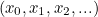then the defining relationship is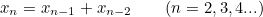(1)

with starting conditions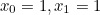. On dividing both sides of (1) by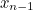we get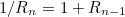where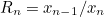, the ratio of successive terms.

As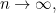we have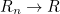so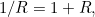giving the equation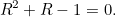This quadratic equation has two roots; the one we need here is obviously between zero and one; it is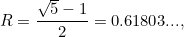(2)

the number known as the golden Ratio.

The number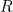has some remarkable properties; for example, it is expressible as a continued fraction: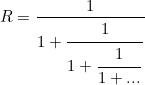(3)

In the theory of chaotic dynamical systems,is recognised as "the most irrational number" between 0 and 1!

The spiral curve shown in the poster is a logarithmic spiral, a curve whose equation in polar coordinates is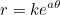where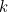and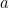are constants. The spiral patterns evident in the sunflower are of this form, and the numbers of spirals going in opposite senses are the consecutive Fibonacci numbers 34 and 55.

The underlying reason for this may be found in many texts; see for example Conway JH and Guy RK The Book of Numbers, Springer-Verlag (1996), chapter 4.

Back to the main article